Next: Elastic medium Up: 3: WHY DISCONTINUITIES? Previous: Characteristics and discontinuities

## Eikonal equation

The characteristic equationdescribes the propagation of a discontinuity in space (x,y,z). Let us assume that equation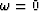can be solved with respect to t: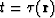and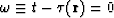. Then we get from equation (20):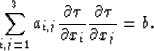(21)

That is exactly the Eikonal equation for a medium with elliptic anisotropy. In the case of isotropy, it follows that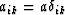and equation (21) is reduced to(22)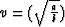.

Let us consider, for example, the linear acoustic equation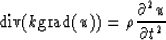orIn this case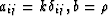and we again get equation (22) at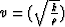.It is very important that the propagation of a discontinuity depends only on senior terms of the equation (18).Next: Elastic medium Up: 3: WHY DISCONTINUITIES? Previous: Characteristics and discontinuities
Stanford Exploration Project
1/13/1998